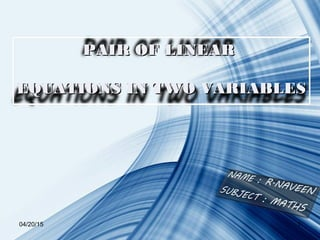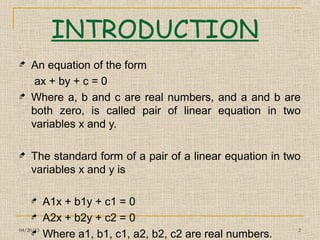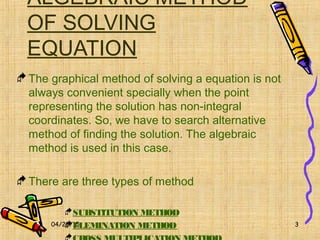Diese Präsentation wurde erfolgreich gemeldet.

# PAIR OF LINEAR EQUATION IN TWO VARIABLE

Anzeige
Anzeige
Anzeige
Anzeige
Anzeige
Anzeige
Anzeige
Anzeige
Anzeige
Anzeige
Anzeige×

1 von 15 Anzeige

# PAIR OF LINEAR EQUATION IN TWO VARIABLE

Power Point Presentation on a PAIR OF LINEAR EQUATION IN TWO VARIABLES, MATHS project...
Friends if you found this helpful please click the like button. and share it :) thanks for watching

Power Point Presentation on a PAIR OF LINEAR EQUATION IN TWO VARIABLES, MATHS project...
Friends if you found this helpful please click the like button. and share it :) thanks for watching

Anzeige
Anzeige

### PAIR OF LINEAR EQUATION IN TWO VARIABLE

1. 1. 04/20/15 1 PAIR OF LINEARPAIR OF LINEAR EQUATIONS IN TWO VARIABLESEQUATIONS IN TWO VARIABLES
2. 2. 04/20/15 2 INTRODUCTION  An equation of the form ax + by + c = 0  Where a, b and c are real numbers, and a and b are both zero, is called pair of linear equation in two variables x and y.  The standard form of a pair of a linear equation in two variables x and y is  A1x + b1y + c1 = 0  A2x + b2y + c2 = 0  Where a1, b1, c1, a2, b2, c2 are real numbers.
3. 3. 04/20/15 3 ALGEBRAIC METHOD OF SOLVING EQUATION The graphical method of solving a equation is not always convenient specially when the point representing the solution has non-integral coordinates. So, we have to search alternative method of finding the solution. The algebraic method is used in this case. There are three types of method SUBSTITUTION METHOD ELEMINATION METHOD
4. 4. 04/20/15 4 SUBSTITUTION METHOD STEP 1 : express y in terms of x from either equation. STEP 2 : substitute this value of y in the other equation resulting it to reduce in an equation in x. solve it for x. STEP 3 : substitute this value of x in the equation used in step 1 to obtain the value of y and get the value of y.
5. 5. 04/20/1504/20/15 55 EXAMPLEEXAMPLE 1)1) x + y =17x + y =17 2x – 3y = 112x – 3y = 11 x + y =17x + y =17 2x – 3y = 112x – 3y = 11 From equation (1)From equation (1) y = 7 – xy = 7 – x Substitute this value of ySubstitute this value of y in equation (2) we getin equation (2) we get 2x – 3(7 – x) = 112x – 3(7 – x) = 11 2x – 21 + 3x = 112x – 21 + 3x = 11 5x – 21 = 115x – 21 = 11 5x = 11 + 215x = 11 + 21 (1) (2) 5x = 325x = 32 x = 32/5x = 32/5 Substitute this value of y in equation (3) we get y = 7 – 32/5 y = 35-32/5 y = 3/5 x = 32/5 y = 3/5 (3)
6. 6. 04/20/1504/20/15 66 ELEMINATION METHODELEMINATION METHOD  step 1step 1 : multiply both the equation by the same: multiply both the equation by the same suitable non-zero constant to make the coefficient ofsuitable non-zero constant to make the coefficient of one variable numerically equal.one variable numerically equal.  STEP 2STEP 2 :: then add or subtract one equation from thethen add or subtract one equation from the other so that one variable gets eliminated.other so that one variable gets eliminated.  STEP 3STEP 3 :: solve the resulting equation in one variable sosolve the resulting equation in one variable so obtained and get the value.obtained and get the value.  STEP 4STEP 4 :: substitute this value of x or y in either of thesubstitute this value of x or y in either of the original equation and get the value of the otheroriginal equation and get the value of the other variables.variables.
7. 7. 04/20/1504/20/15 77 EXAMPLEEXAMPLE (1) 5x + 3y = 70(1) 5x + 3y = 70 3x – 7y = 603x – 7y = 60 5x + 3y = 705x + 3y = 70 3x – 7y = 603x – 7y = 60 multiplying equation (1) by 3multiplying equation (1) by 3 multiplying equation (2) by 5multiplying equation (2) by 5 15x + 9y =21015x + 9y =210 15x – 35y = 30015x – 35y = 300 subtracting equation (4) fromsubtracting equation (4) from equation (3) we getequation (3) we get 15x + 9y =21015x + 9y =210 15x – 35y = 30015x – 35y = 300 (1) (2) 44y = -90 44y = -90 y = -90/44 y = -45/22 Substituting this value of y in equation (1) we get 5x + 3(-45/22) = 70 5x – 135/22 = 70 5x = 70 + 135/22 5x = 1540 + 135/22 5x = 1675/22 x = 335/22 x = 335/22 y = -45/22
8. 8. 04/20/1504/20/15 88 CROSS-MULTIPLICATIONCROSS-MULTIPLICATION METHODMETHOD STEP 1STEP 1 :: draw a diagram as followdraw a diagram as follow XX YY 11 b1b1 c1c1 a1a1 b1b1 b2b2 c2c2 a2a2 b2b2  STEP 2 : then write the equation as follows X Y 1 B1c2-b2c1 c1a2-c2a1 a1b2- a2b1  STEP 3 : if a1b2 – a2b1 = 0 find x and y as follows b1c2-b2c1 c1a2-c2a1 = = X = Y =
9. 9. 04/20/15 9 EXAMPLE 1) 11x + 15y + 23 = 0 7x – 2y -20 = 0 X Y 1 15 23 11 15 -2 -20 7 -2 X Y (15)(-20)-(-2)(23) (23)(7)-(120)(11) 1 (11)(-2)-(7)(15) =
10. 10. 04/20/15 10 X Y 1 -300+46 161+220 -22-105 X Y 1 -254 381 -127 X = -254/-127 = 2 Y = 381/-127 = 3 X = 2 Y = 3 = = = =
11. 11. 04/20/15 11 GRAPHICAL METHOD OF SOLVING EQUATION  graphical representation of linear equation in two variables is a straight line. As a straight line consist of an infinite no of points lying on it.  Given a pair of linear equation in two variables representing two straight lines taken together, only one of the following three possibilities can occur  The two lines intersect at one point.  The two lines are parallel.  The two lines are coincident.
12. 12. 04/20/15 12  The two lines intersects at one point  the two lines are parallel  the two lines are coincident
13. 13. 04/20/15 13 EXAMPLE 1) Sangeeta has socks and hand kerchiefs which are together 40 in no. If she has 5 less handkerchiefs and 5 more socks, the number of socks becomes four times the number of hand kerchiefs. Represent this situation in algebraically and graphically. X + y = 40 X + 5 = 4(y -5) X + 5 = 4y – 20 X – 4y = -25 For equation (1) X + y = 40 Y = 40 – x For equation (2) X – 4y = -25 4y = x + 25 X 20 40 Y 20 0 (1) (2) X 15 55 Y 10 20
14. 14. 04/20/15 14 -80 -70 -60 -50 -40 -30 -20 -10 0 10 20 30 40 50 60 70 80 -10 -20 -30 -40 -50 -60 -70 60 50 40 30 20 10 70 X – 4y = -25 X + y = 40 (55,20) (20,20) (15,10) (40.0) (27,13)
15. 15. 04/20/15 15